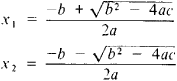Also found in: Dictionary, Thesaurus, Acronyms, Wikipedia.

(mathematics)
Any second-degree polynomial equation.
McGraw-Hill Dictionary of Scientific & Technical Terms, 6E, Copyright © 2003 by The McGraw-Hill Companies, Inc.
The following article is from The Great Soviet Encyclopedia (1979). It might be outdated or ideologically biased.

an equation of the form ax2 + bx + c = 0, where a, b, and c are any number and are called the coefficients of the equation. A quadratic equation has two roots, which are found by the formulasThe expression D = b2 − 4ac is called the discriminant of the quadratic equation. If D > 0, then the roots of the quadratic equation are real and unequal; if D < 0, then the roots areconjugate complex numbers; if D = 0, then the roots are real andequal. The Vièta formulas x1 + x2 = − b/a and x\x2 = c/a link the roots and coefficients of a quadratic equation. The left-hand side of a quadratic equation can be expressed in the form α (xx2) (xx2). The function y = ax2 + bx + c is called a quadratic trinomial, and its graph is a parabola with the vertex at the point M (−b/2a; cb2/4a) and axis of symmetry parallel to the. y-axis; the direction of the branches of the parabola coincides with the sign of a. The solution of the quadratic equation was already known in geometric form to ancient mathematicians.